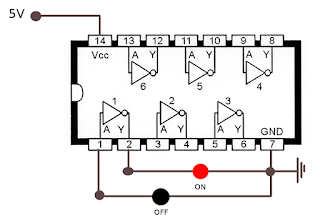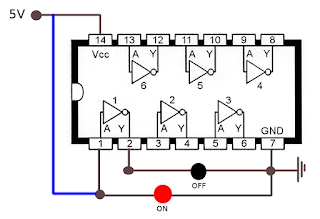## 24 October 2016

### Inverter Circuit with an 74HC04 Chip

Lately, you'll have realised I'm writing about electronics like UART connections of an Orange router or how to see the booting process of broadband routers. Therefore, I have used words like multimeter, oscilloscopio, ground, volts, etc in previous posts. However, this time, I want to go beyond and I have bought a couple of inverters with some leds and a breadboard to make some experimental circuits. At first, in this post, we'll see how the 74HC04 inverter works and how to test it.

An inverter is a chip that it's be able to change the logic state of an input to the opposite state. For example, if the input is a HIGH logic signal, the output is a LOW logic signal, and if the input is a LOW logic signal, the output is a HIGH logic signal. This is a basic and necessary chip when we want the opposite state of an input state.

The 74HC04 hex inverter chip has 6 gates where the logic signal output by an inverter gate is the opposite of the logic signal fed into the gate. In addition, we can see in the 74HC04 datasheet that the A's of each gate are the inputs and the Y's of each gate are the outputs, meaning Y is the opposite logic signal of A.

Once we know how it works, it's time to test it. I've built a small circuit with an inverter chip and two leds. One led is connected to the input (A) and the second led is connected to the output (Y) with the goal of testing the first gate. Therefore, one led is connected between the first pin (A) and the seventh pin (GND) and the other led is connected between the second pin (Y) and the seventh pin (GND). I've also fed the inverter chip through the 14 pin with 5 volts and I've connected it to the ground as well through the seventh pin. What should there be the result? The led number 1 turned off because it's not being fed and the led number 2 turned on because the opposite of a LOW logic signal in the first pin is a HIGH logic signal for the second pin, where the led number 2 is connected. It is represented in the next diagram:Led 1 turned off and Led 2 turned on

On the other hand, if the first pin (A) has a HIGH logic signal with 5V, we'll see that the led number 1 is turned on while the led number 2 is turned off. Why? Because the led number 1 is being fed and the led number 2 has the opposite, not being fed, as a result, turned off. It is represented in the next diagram:Led 1 turned on and Led 2 turned off

This is an easy way to test each gate of our inverter chips and especially to understand how it works. Next, we have a video I recorded.

Regards my friends, drop me a line with the first thing you are thinking.